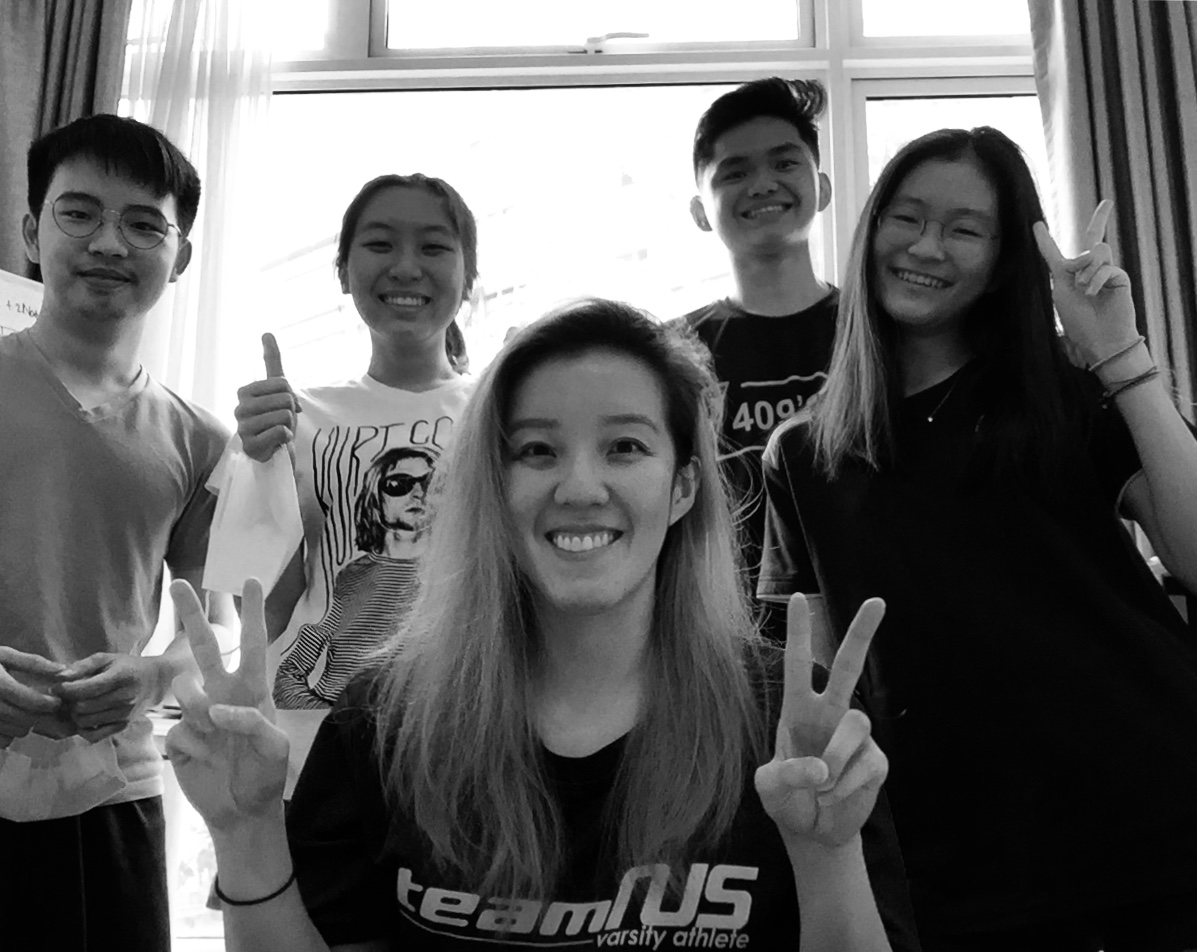# H2 Level Math Tuition### Get Started at Pamela’s Place

A Chemistry, Biology & Maths Specialist for ‘A’ Level & ‘O’ level .

PAMELA’S PLACE is the fruit of my academic journey. I’ve been a student before, I’ve known a subject to be challenging and had become unmotivated to excel. However, we all have our strengths, and I have perfected mine over the years so I can help you focus on yours. This is my passion and labour. My students are my results and why I am committed to helping more people wholly towards grasping & aceing the subject.

### Study Smart, Top the Chart!

What is A Level H2 Maths?

The H2 Math was created by the Ministry of Education (MOE) to prepare students for a multitude of university courses that require a mathematical basis, such as some sciences including chemistry and physics, pure mathematics or math for educators, and engineering. It improves numerical reasoning and critical thinking skills, which are necessary for more in-depth math studies.

H2 Math is also vital for more than just passing the A-level Maths examinations. It also provides useful information that may be used for a variety of university academic subjects. Many of the abilities and problem-solving principles taught in JC H2 math tuition can be applied to real-world situations and difficulties addressed by the various academic disciplines.

What is A Level H2 Maths Tuition About?

As expected from JC level subjects, JC H2 Maths are more demanding than the previous mathematics subjects the students have gone through. Most topics included in H2 Maths aim to hone the students’ problem solving skills as well as confidence in explaining his solution and understanding of the mathematical concepts. Thus, good practice is necessary to excel in H2 Maths. One way for that to be achieved is through the help of A-level Math Tuition in Singapore.

JC Math subjects are in different levels of difficulty as compared to the previous Maths a student may have taken during secondary school. Not to mention, the end goal of A-level JC H2 Math Tuition is not just to pass the A levels math exams but to allow them to excel in the future degree he or she plans to pursue in university.

I want to help my tutees not only on practical examination strategies so that they may get an A on their H2 Math exams, but also to enjoy and create an interest in mathematics. Pamela’s Place also recognises that practice is the key towards excellence.

As a result, we enact a wide selection of H2 Math tests in order to help them acquire the skills to solve difficult problems that they may encounter. Taking H2 Math tuition from Pamela’s Place will prepare you in your future learning journey!

What is A Level H2 Maths Syllabus about?

A-level H2 Maths Syllabus is designed to help the students prepare for the A-level Math Exam, especially the JC H2 Maths topics. Since H2 Maths are often difficult to master, it will take a considerable amount of time and a comprehensive outline for a progressive learning process. Some of the key lessons included in the syllabus are:

• Understanding functions and graphs
• A more in-depth knowledge about sequence and series
• Introduction to complex numbers
• Understanding Calculus
• In-depth Learning of Probability and Statistics

FAQs about A Level H2 Maths

Is H2 math hard?

JC H2 Maths can be quite difficult as the topics are more complex and advanced since the A-level Math exam is meant to test the learner’s capabilities to pursue further learnings in universities and colleges.

How long is H2 math paper?

Three hours are allotted for the JC H2 papers in the GCE examination. The paper consists of objective questions as well as open-ended questions to test the student’s understanding and numerical reasoning skills.

Do u need H2 math for medicine?

The National University of Singapore doesn’t require A-level Maths or JC H2 Mathematics for taking courses related to medicine. However, some international universities might require these units so it is safe to pursue the A-level Math exam if you wish to study medicine abroad.

What topics are in A level maths?

The syllabus used for A-level Math Tuition in Singapore includes topics such as Graphs and Functions, Series and Sequence, Vectors, Introduction to Complex Numbers, Calculus, and Probability and Statistics.

Is H2 math important?

H2 level Mathematics is crucial in such a way that you have to pass the A-level Math examination which includes these topics if you wish to pursue courses that are associated with mathematics in universities and colleges.

What are the most difficult in A levels?

Most students will argue that A-level Mathematics is the hardest subject among the ones included in the GCE exam. That is why, it is crucial for students to take an A-level Math tuition for early review and preparation before the actual exam.